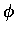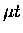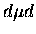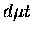Next: Epithermal molecular formation Up: Measurement of resonant molecular Previous: Measurement of resonant molecular

## Cycling measurements in D/T mixture

In conventional experiments [61,62,63,64,65,,67,68,69], the molecular formation rates are determined from the measurements of the cycling rate, which is, whereis the mean time between the 14 MeV neutrons from fusions catalyzed by the same muon.

In this method, the cycling rates, measured at various target conditions (such as the tritium concentration ct, temperature T, and the density), are fitted to an expression describing the kinetics model. Molecular formation rates, together with quantities such as thetransfer rateand q1s, are the fitting parameters. A simplified expression forreads :(13)whereis the fraction of,transition with the spin flip rate, andis a correction factor taking into account thefusion time, withandbeingformation and fusion rates, respectively (noteformation is non-resonant, hence assumed to be independent of F). An improved formula would include a further correction term due to the muon recycling inandbranches without producing the 14 MeV neutrons, which depends on parameters likeformation and spin flip rates.

The molecular formation rate depends not only on thehyperfine state F, but also on the target molecular species. Thus,consists of different components(14)

which have to be disentangled in the fit. Often, assumptions have to be made in actual fits with guidance from theory. For example, at low temperatures all the terms inexceptare assumed to be zero [67,22]. We shall come back to the implications of these assumptions later in this thesis.

As Cohen points out , further complication in the conventional method comes from the lack of precise characterization of the parameter q1s (the probability that the muon reaches the ground state ofbefore transferring to a triton). Its behavior suggested by the cycling fit is in large disagreement with theoretical expectations of its ct anddependence. Independent measurements of qpd1s for pd transfer are emerging , but no direct information is yet available for the dt case.

Furthermore, the recent suggestion by Froelich and Wallenius of a resonant side-path in D/T cycling [44,49] (see Section 1.2.4) could have significant impact in the extraction of theformation rate from the cycling rate.

Finally, it should be noted that in these analyses using single rates, the energy dependences of the processes are averaged out.Next: Epithermal molecular formation Up: Measurement of resonant molecular Previous: Measurement of resonant molecular